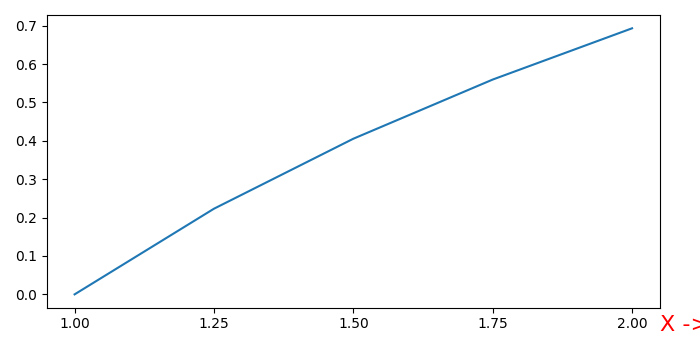# How to set my xlabel at the end of X-axis in Matplotlib?

To set the xlabel at the end of X-axis in matplotlib, we can take the following steps −

• Create data points for using numpy.

• Using subplot() method, add a subplot to the current figure.

• Plot x and log(x) using plot() method.

• Set the label on X-axis using set_label() method, with fontsize=16, loc=left, and color=red.

• To set the xlabel at the end of X-axis, use the coordinates, and y.

• To display the figure, use show() method.

## Example

import numpy as np
from matplotlib import pyplot as plt
plt.rcParams["figure.figsize"] = [7.00, 3.50]
plt.rcParams["figure.autolayout"] = True
x = np.linspace(1, 2, 5)
ax = plt.subplot()
ax.plot(x, np.log(x))
ax.set_xticks(x)
label = ax.set_xlabel('X ->', fontsize=16, loc="left", c="red")
ax.xaxis.set_label_coords(1.0, -0.025)
plt.show()

## Output